• c++数据结构小助手 : 可视化树与图工具 基于 graphics.h； 从文件 graph.txt 读图 格式如下： 命名：graph.txt 第一行，两个整数，n个点和m条边，边全都是有向边 第二行到第m+1行 每行三个整数 a b c 表示从a到b有一...

c++数据结构小助手 : 可视化树与图工具

基于 graphics.h；
从文件 graph.txt 读图
格式如下：
命名：graph.txt
第一行，两个整数，n个点和m条边，边全都是有向边
第二行到第m+1行
每行三个整数
a b c
表示从a到b有一条权重为c的边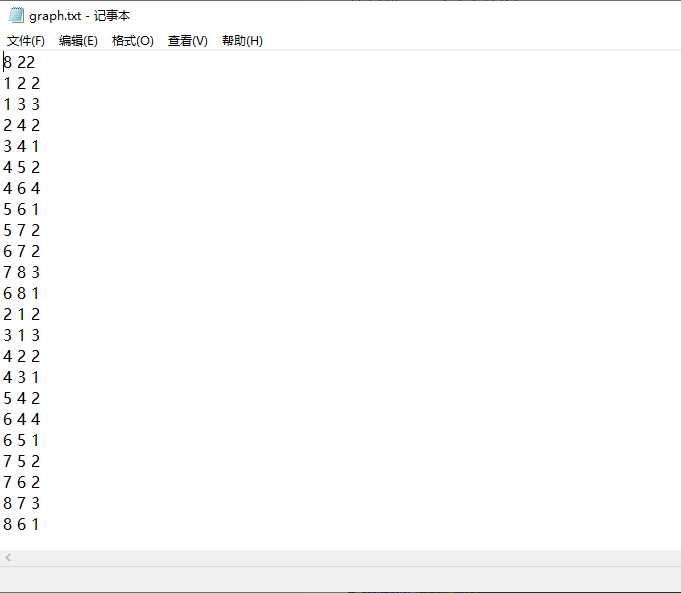无向边就是两条有向边

效果：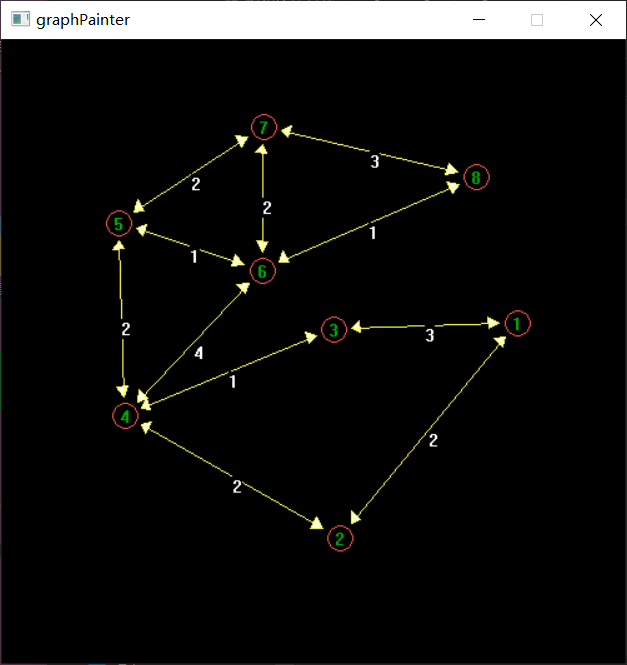左键点击点可以拖动改变布局

图中箭头是算出来的

#include <iostream>
#include <stdio.h>
#include <graphics.h>
#include <unordered_map>
#include <vector>
#include <queue>
#include <cmath>
#include <cstring>
#include <conio.h>
#include <functional>
#include <stdio.h>
#include <io.h>
#include <string>
#include <fstream>
#include <sstream>
#include<time.h>

/*
从文件读取

本人平时不太喜欢写注释，特别是在写一些比较小的程序的

左键点击图中的点有惊喜

*/

#define MAX_LINE 1024
#define MAXR 100
#define MINR 1

using namespace std;

#define WINDOWW 500
#define WINDOWH 500
#define X_AXIS WINDOWH/2
#define Y_AXIS WINDOWW/2
#define MIDW WINDOWW/2
#define MIDH WINDOWH/2
#define PI 3.1415926
#define VER_SIZE 10

#define MAX_VERS 2000

typedef pair<int, int> pii;
typedef pair<int, pii> pi_ii;

int n, m;

double flag = (degree < 0) ? -1.0 : 1.0;
if (degree < 0){
degree = degree*-1.0;
}
double res = flag*(degree*PI) / 180;
return res;
}

void intToChar(int i, TCHAR res[]){ // int转char
char t;
int p = 0, q = 0;
while (i){
t[p++] = i % 10 + '0';
i /= 10;
}
for (int i = p - 1; i >= 0; i--){
res[q++] = t[i];
}
res[q] = '\0';
}

bool drawArray(int x1, int y1, int x2, int y2, double breath = 15){ // 画箭头
int xa, ya, xb, yb, xt, yt;

if (x1 == x2){
xt = x1;
if (y1 > y2){
y1 -= breath;
y2 += breath;
yt = y2 + breath / 2;
}
else{
y1 += breath;
y2 -= breath;
yt = y2 - breath / 2;
}
xa = xt - breath / 2;
ya = yt;
xb = xt + breath / 2;
yb = yt;
}
else{
double k = (double)(y1 - y2) / (double)(x1 - x2);
double dx, dy;
dx = breath / sqrt(1 + k*k);
dy = fabs(k)*dx;
if (k >= 0){
if (y1 < y2){
x1 += dx;
y1 += dy;
x2 -= dx;
y2 -= dy;
xt = x2 - dx / 2;
yt = y2 - dy / 2;
}
else{
x2 += dx;
y2 += dy;
x1 -= dx;
y1 -= dy;
xt = x2 + dx / 2;
yt = y2 + dy / 2;
}
xa = xt + dy / 3;
ya = yt - dx / 3;
xb = xt - dy / 3;
yb = yt + dx / 3;
}
else{
if (y1 > y2){
x1 += dx;
y1 -= dy;
x2 -= dx;
y2 += dy;
xt = x2 - dx / 2;
yt = y2 + dy / 2;
}
else{
x2 += dx;
y2 -= dy;
x1 -= dx;
y1 += dy;
xt = x2 + dx / 2;
yt = y2 - dy / 2;
}
xa = xt - dy / 3;
ya = yt - dx / 3;
xb = xt + dy / 3;
yb = yt + dx / 3;
}

}
POINT pts[] = { { x2, y2 }, { xa, ya }, { xb, yb } };
fillpolygon(pts, 3);
line(x1, y1, x2, y2);
return true;
}

unordered_map<int, pii> initVerXY(int vertices){
double D_degree = 360.0 / (double)vertices;
double degree = 0;
unordered_map<int, pii> vers;

for (int i = 1; i <= vertices; i++){
int verY, verX;

if ((0 <= degree&&degree <= 90) || (270 < degree&&degree <= 360)){
if (-1 <= k && k <= 1){
verX = (WINDOWW - MIDW) / 2;
verY = k*verX;
}
else{
verY = (WINDOWH - MIDH) / 2;
if (k < 0) verY = -verY;
verX = verY / k;
}
}
else{
if (-1 <= k && k <= 1){
verX = (MIDW - WINDOWW) / 2;
verY = k*verX;
}
else{
verY = (MIDH - WINDOWH) / 2;
if (k < 0) verY = -verY;
verX = verY / k;
}
}
degree += D_degree;

int num1 = rand() % (MAXR - MINR) + MAXR;
int num2 = rand() % (MAXR - MINR) + MAXR;
int num3 = rand() % (MAXR - MINR) + MAXR;
int num4 = rand() % (MAXR - MINR) + MAXR;
verX = verX + num1 - num3;
verY = verY + num2 - num4;

vers[i] = { verX + MIDW, verY + MIDH };
}

return vers;
}

bool drawGraph(unordered_map<int, pii> vers, priority_queue<pi_ii> sides){

for (int i = 1; i <= vers.size(); i++){
setcolor(LIGHTRED);
TCHAR s;
intToChar(i, s);
int verX = vers[i].first, verY = vers[i].second;
circle(verX, verY, VER_SIZE);
setcolor(WHITE);
settextcolor(GREEN);
outtextxy(verX - 4, verY - 7, s);
settextcolor(WHITE);
}

while (sides.size()){
pi_ii t = sides.top();
sides.pop();
int verA = t.second.first;
int verB = t.second.second;
int len = t.first;
setcolor(YELLOW);
drawArray(vers[verA].first, vers[verA].second, vers[verB].first, vers[verB].second);
setcolor(WHITE);
TCHAR s;
intToChar(len, s);
outtextxy(vers[verA].first + vers[verB].first >> 1, vers[verA].second + vers[verB].second >> 1, s);
}
return true;
}

template<class out_type, class in_value>
out_type convert(const in_value & t)
{
stringstream stream;
stream << t;// 向流中传值
out_type result;// 这里存储转换结果
stream >> result;// 向result中写入值
return result;
}

bool checkIfGetVer(int vx, int vy, int mx, int my){
double d;
d = sqrt((double)(vx - mx)*(vx - mx) + (double)(vy - my)*(vy - my));
if (d < VER_SIZE) return true;
return false;
}

void dragMove(unordered_map<int, pii> vers, priority_queue<pi_ii> sides){
MOUSEMSG clickdown;
MOUSEMSG down;
while (true){
clickdown = GetMouseMsg();

switch (clickdown.uMsg){
case WM_LBUTTONDOWN: // left click

for (int i = 1; i <= vers.size(); i++){
if (checkIfGetVer(vers[i].first, vers[i].second, clickdown.x, clickdown.y)){
int flag = 0;

while (true){
down = GetMouseMsg();
switch (down.uMsg){
case WM_MOUSEMOVE:
BeginBatchDraw(); // double huan chong
cleardevice();
vers[i].first = down.x;
vers[i].second = down.y;
drawGraph(vers, sides);
EndBatchDraw();
break;
case WM_LBUTTONDOWN:
flag = 1;
break;
}
if (flag)break;
}
}
}
break;
case WM_LBUTTONUP:
break;
}

}

}

int main(){
srand(time(0));
char buf[MAX_LINE];
string buff;
FILE *fp;
int len;

if ((fp = fopen("graph.txt", "r")) == NULL)
{
exit(1);
}
fgets(buf, MAX_LINE, fp);
len = strlen(buf);
buf[len - 1] = '\0';
buff = buf;
stringstream sstream;
sstream << buff;

string t;
sstream >> t;
n = convert<int>(t);
//printf("%d ", n);
sstream >> t;
m = convert<int>(t);
//printf("%d\n", m);

priority_queue<pi_ii> sides;

while (fgets(buf, MAX_LINE, fp) != NULL)
{
stringstream sstream;
string t;
len = strlen(buf);
buf[len - 1] = '\0';
int a, b, c;
buff = buf;
sstream << buff;
sstream >> t;
a = convert<int>(t);
sstream >> t;
b = convert<int>(t);
sstream >> t;
c = convert<int>(t);
sides.push({ c, { a, b } });
printf("起点：%d，终点：%d，权重：%d\n", a, b, c);
}
unordered_map<int, pii> vers;
vers = initVerXY(n);
initgraph(WINDOWW, WINDOWH);
//circle(0, 0, 10);

drawGraph(vers, sides);
dragMove(vers, sides);
//while (1);
// printGraph(n, sides);

// dragMove();

closegraph();

getchar();
getchar();
return 0;
}

展开全文c++ 数据结构 可视化
• C++数据结构

2021-01-28 11:51:12
C++中的数组存储相同类型数据项，而结构（struct）时一种用户自定的数据类型，允许用户存储不同类型数据项。 定义结构 struct 语句格式 struct type_name { member_type1 member_name1; member_type2 member_name2; ...

C++中的数组存储相同类型数据项，而结构（struct）时一种用户自定的数据类型，允许用户存储不同类型数据项。

定义结构

struct 语句格式

struct type_name {
member_type1 member_name1;
member_type2 member_name2;
member_type3 member_name3;
.
.
} object_names;

//例如
struct Books
{
char title;
char auther;
char subject;
int book_id;
} book;

访问结构成员

成员访问运算符（.）。

cin >> book.title;

cout << book.title <<endl;

结构作为函数参数

传参方式与其他类型变量或指针类似。

void whoistheauthor ( struct Books book )
{
cout << book.author << endl;
}

指向结构的指针

struct Books *struct_ptr;
struct_ptr = &book;

//为了使用该指针所指结构变量的成员，要用到->运算符
struct_ptr->title;

typedef 关键字

typedef 加在 类型关键字 之前 可以为该类型取一个“别名”， 这同样可以为 struct 服务，省去每次定义该 结构变量 时的繁琐

typedef struct Books
{
char title;
char auther;
char subject;
int book_id;
}Books;

Books Book1, Book2;

//其他类型示例
typedef long int *pint32;

pint32 x, y, z;

展开全文c++
• 链表是一种物理存储单元上非连续的存储结构数据元素的逻辑顺序是通过链表中的指针链接实现的 链表由一系列结点组成，一个是存储数据元素的数据域，另一个是存储下一个结点地址的指针域。 数组和链表区别： STL中...

基本概念

功能：将数据进行链式存储
链表是一种物理存储单元上非连续的存储结构，数据元素的逻辑顺序是通过链表中的指针链接实现的
链表由一系列结点组成，一个是存储数据元素的数据域，另一个是存储下一个结点地址的指针域。

数组和链表区别：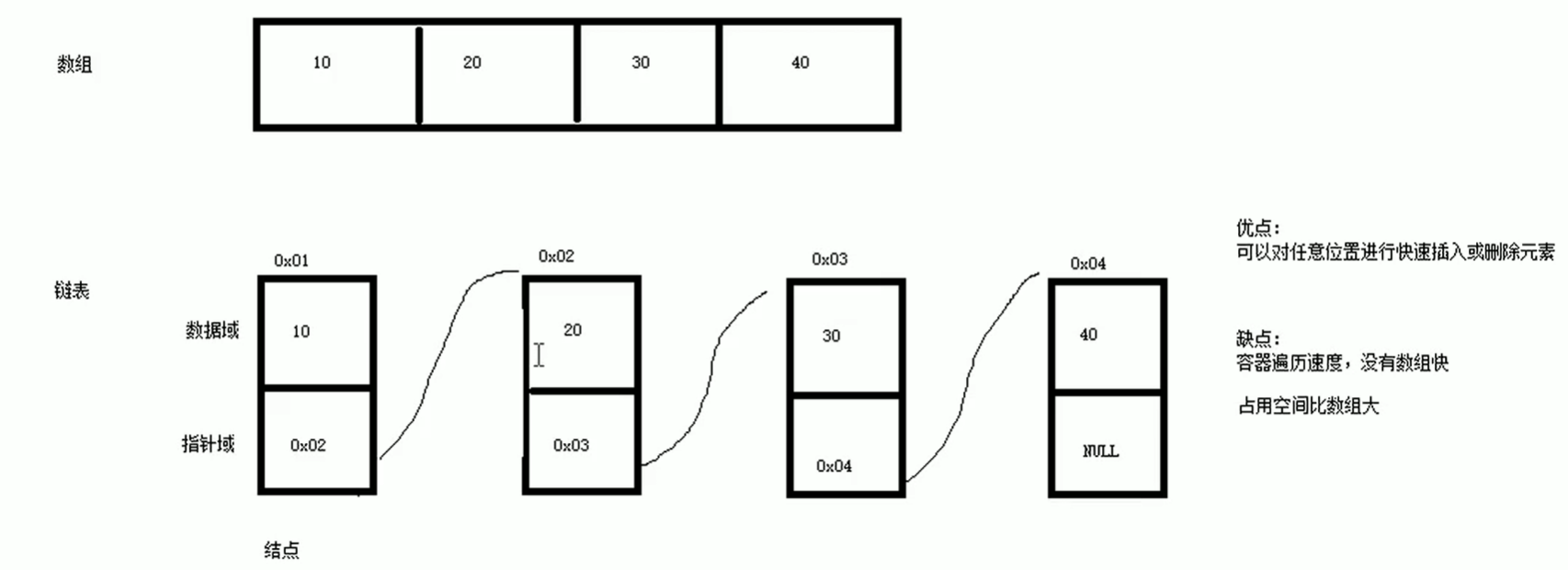STL中的链表是一个双向循环链表：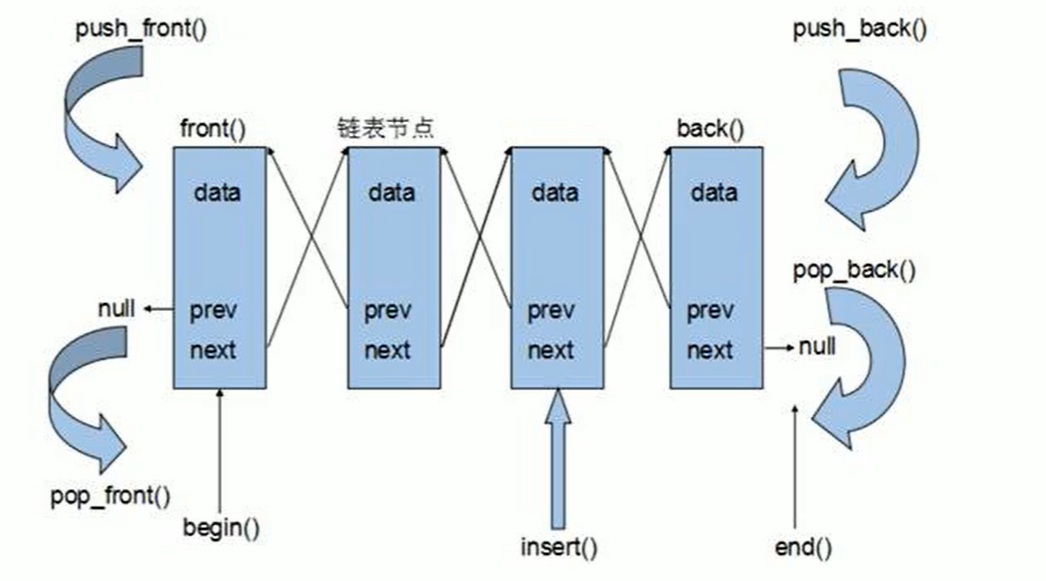特点是：

1）指针域不仅有指向后向结点的next 还有指向前向结点的prev
2）最后结点的next不是指向Null，而是第一个结点；第一个结点的prev不是指向Null，而是最后一个结点；
3）push_front()、pop_front()、begin()、front()、back()、pop_back()、push_back()、end()
4）迭代器为双向迭代器，不能随机访问（这个要牢记 就是it只能++ --)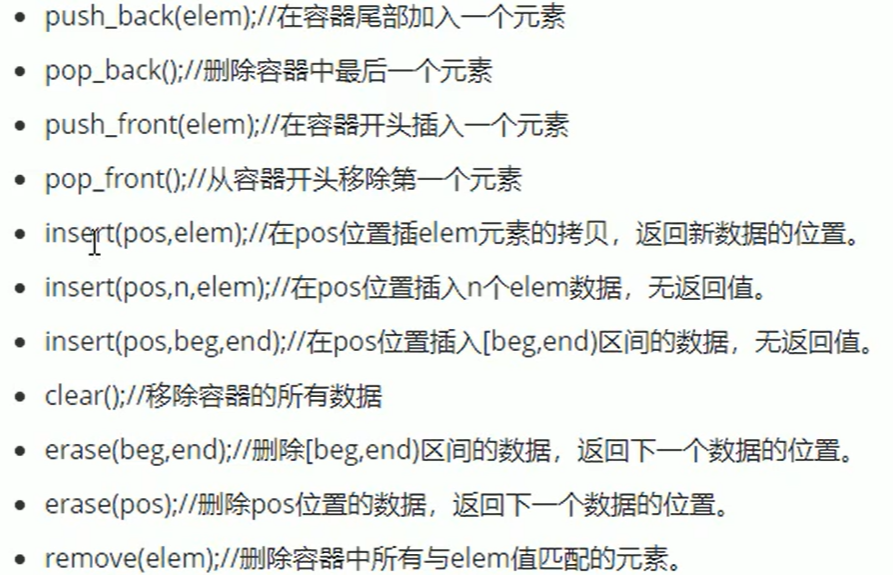展开全文链表 c++

链式结构
链式数据结构

typedef struct LNode {

ElemType data;
struct LNode *next;

链队列图片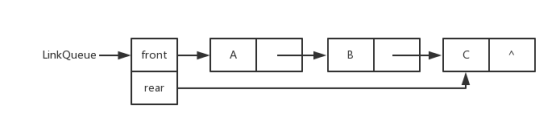线性表的链式表示

单链表图片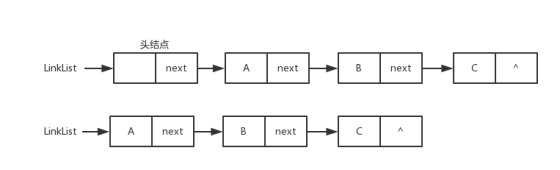双向链表图片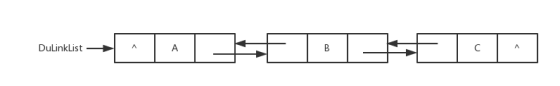循环链表图片

展开全文• C++数据结构】二叉树初始化以及遍历 先放代码： #include <iostream> #include <string> using namespace std; typedef int ElemType; struct BiTree{ ElemType data; BiTree *left; BiTree * ...c++ 二叉树 数据结构
• C++数据结构算法 一、提出问题 1、什么是函数重载？ 答：具有不同形参列表的同名函数称为函数重载，编译系统根据实参的类型来选择函数，这个过程称为重载解析。 2、什么是运算符重载？ 答：在实际应用中，我们常常要...c++ 算法 数据结构
• } //向队尾插入一个数据元素 int EnQueue(SqQueue &sq, ElemType x) //循环队列操作 { if ((sq.rear + 1) % MaxSize == sq.front) //循环队列判断队满条件：(rear+1)%MaxSize==front return 0; sq.rear = (sq.rear ...数据结构 c++ c语言
• C++数据结构——BF算法

千次阅读 2021-04-02 08:55:10
/* BF算法 */ #include <iostream> #include <string> using namespace std; int main() { string s1,s2; cin>>s1>>s2; int i=0,j=0,start=0; while (s1[i]!='\0'&... {
• 许多实际问题抽象出来的数据结构往往是二叉树形式，即使是一般的树也能简单地转换为二叉树，而且二叉树的存储结构及其算法都较为简单，因此二叉树显得特别重要。二叉树特点是每个结点最多只能有两棵子树，且有左右之...链表 数据结构 c++
• C++题库 这里仅列出题目名称，需要的同学与我联系szujrjzh@outlook.com T1 DS图—最小生成树 2.0.cpp T1 DS二叉树——Huffman编码与解码(不含代码框架).cpp T2 图综合练习–构建邻接表.cpp T5 DS图—图的连通分量....c++ c语言 数据结构
• C++数据结构 栈实现中缀表达式计算 一、问题描述 算符优先法：根据运算符优先关系来实现对表达式进行编译执行，为简单起见只讨论四则运算 优先级表格如下： 二、算法描述 设置两个栈，操作数栈opnd和操作符栈optr ...c++ 算法 数据结构
• C++中常用的数据结构

千次阅读 2021-01-25 23:14:51
一直以来虽然写的都是C++的代码但是基本上用的都是C的数据结构。这次实现一个相对大型的C++项目，发现了很多很有用的数据结构，通过使用这些数据结构，可以极大程度的提高开发效率。所以计划逐渐整理下C++数据结构...数据结构 c++ 算法
• C/C++ 数组允许定义可存储相同类型数据项的变量，但是结构C++ 中另一种用户自定义的可用的数据类型，它允许您存储不同类型的数据项。 结构用于表示一条记录，假设您想要跟踪图书馆中书本的动态，您可能需要跟踪每...c++
• C++数据结构---priority_queue（优先队列，堆）1. 什么是priority_queue（优先队列，堆)2. 如何使用 1. 什么是priority_queue（优先队列，堆) 普通的队列的性质是先进先出，元素在队列尾加入，在队头弹出。但是在...
• /* 线性表——顺序查找 */ #include<iostream> #include<string> using namespace std; const int maxsize=100; class LinkSearch { private: int data[maxsize]; int length;...LinkSearch:数据结构 算法
• C++数据结构——散列表（哈希表）

多人点赞 热门讨论 2021-06-22 20:12:59
定义：散列表（Hash table，也叫哈希表），是根据关键码值(Key value)而直接进行访问的数据结构。也就是说，它通过把关键码值映射到表中一个位置来访问记录，以加快查找的速度。这个映射函数叫做散列函数，存放记录...数据结构 算法 哈希表 C++
• /* 二路归并排序（递归实现） */ #include <iostream> using namespace std; int length=12; int *data; void Merge(int first1,int last1,int last2) { int *temp=new int[length];...=last2)数据结构 c++ 排序算法
• 本书使用Python 和C++两种编程语言来介绍数据结构。全书内容共15 章。书中首先介绍了抽象与分析、数据的抽象等数据结构的基本原理和知识，然后结合Python 的特点介绍了容器类、链式结构和迭代器、堆栈和队列、递归、...
• #include <iostream> #include <memory> #include <vector> #include <unordered_map> #include <string> #include <queue> template <class T>...tempc++ 数据结构 开发语言
• 实验原理 代码思路 使用两个顺序栈编程，一个顺序栈用来存储操作数，一个顺序栈用来存储操作符 关键为表达式求值函数的编写 对该函数，循环... } 运行结果 参考链接 b站up主：laura柳 b站up主:跟懒猫老师快乐数据结构c++ 数据结构 算法
•算法 数据结构 c++
• 1.单纯交换节点内部的值： ListNode * swapPairs(ListNode * head) { // write your code here ListNode* p = head; while(p != NULL && p->next != NULL){ ListNode* q = p->... q-
• 现在工作上常用C++，也是为了业余时间锻炼思维，开始回顾下数据结构，在B站上找不到太像样的基于C++数据结构课程，好不容易找到了来自黑马程序员的一个号称基于C++数据结构课程，结果打开一看，基本是就是纯C...
•链表 数据结构 c语言 c++
• 元旦前后利用几天时间完成了数据结构课程设计，学期初计划搞一个精美 些的课程设计，最后还是一拖再拖，到最后只来得及实现些基本功能。 团队协作完成，所以代码风格不尽相同。 另外才疏学浅，若有错误恳请不吝赐教...编程语言 c++ 数据结构
• } 查找方式为“从右到左查找+监视哨”的算法分析： 二：有序表的二分查找 如果表中的数据都是排列好的，那么就无需从头开始找，就像翻字典一样。 代码实现： #include using namespace std; int binSearch_1(int* a...算法 数据结构 c++
• C++基础数据结构 | 树（Tree） 144. 二叉树的前序遍历 C++实现二叉树的前中后层序遍历（递归和迭代） 迭代和递归 94. 二叉树的中序遍历 ...
• C++基础数据结构 | 链表（linked list） 206. 反转链表 92. 反转链表 II 25. K 个一组翻转链表 复用92. 链表 II 2. 两数相加 carry位加一 ...链表 数据结构 c++ 指针
• c++ var a = Enumerable.Repeat(1, 10).ToList(); vector a(10,1) var a = new List(b); vector a(b); a.InsertRange(0,b.AsEnumerable().Take(3)); a.assign(b.begin(), b.begin()+3); a.assign(4,2)...
• 第一章 编写一个程序解决选择问题，令k=N/2.得到所编程序对于N的不同值的运行时间 （语法方面很多不熟悉，所以做了相关的笔记注释） ...//为了使用日期和时间相关的函数和结构，需要在 C++ 程序中引用 <c++ 算法 数据结构...

c++数据结构c++ 订阅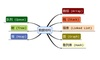数据结构 订阅